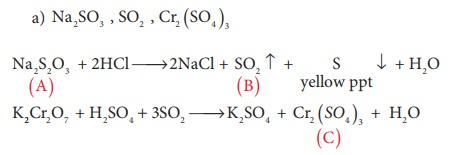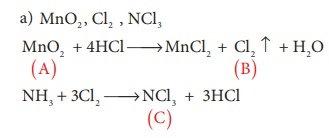Home | | Chemistry 12th Std | Transition and Inner Transition Elements: Choose the best answer

# Transition and Inner Transition Elements: Choose the best answer

Chemistry : Transition and Inner Transition Elements: Choose the best answer with Answers, Solution and Explanation

Chemistry : Transition and Inner Transition Elements

EVALUATION

1. Sc( Z=21) is a transition element but Zinc (z=30) is not because

a) both Sc3+ and Zn2+ ions are colourless and form white compounds.

b) in case of Sc, 3d orbital are partially filled but in Zn these are completely filled

c) last electron as assumed to be added to 4s level in case of zinc

d) both Sc and Zn do not exhibit variable oxidation states

2. Which of the following d block element has half filled penultimate d sub shell as well as half filled valence sub shell?

a) Cr

b) Pd

c) Pt

d) none of these

Solution

Cr  [Ar] 3d5 4s1

3. Among the transition metals of 3d series, the one that has highest negative ( M2+ /M) standard electrode potential is

a) Ti

b) Cu

c) Mn

d) Zn

4. Which one of the following ions has the same number of unpaired electrons as present in V3+?

a) Ti3+

b) Fe3+

c) Ni2+

d) Cr3+

5. The magnetic moment of Mn2+ ion is

a) 5.92BM

b) 2.80BM

c) 8.95BM

d) 3.90BM

Solution

Mn2+ => 3d5 contains 5 unpaired electrons

n=5 ; … = √n(n+2) BM

= √[5(5+2)] = √35 = BM

6. Which of the following compounds is colourless?

a) Fe3+

b) Ti4+

c) Co2+

d) Ni2+

Solution

Ti4+ contains no unpaired electrons in d orbital, hence no d-d transition.

7. the catalytic behaviour of transition metals and their compounds is ascribed mainly due to

a) their magnetic behaviour

b) their unfilled d orbitals

c) their ability to adopt variable oxidation states

d) their chemical reactivity

8. The correct order of increasing oxidizing power in the series

a) VO2+ < Cr2O72−< MnO4

b) Cr2O72− < VO2+ < MnO4

c) Cr2 O72− < MnO4  < VO2+

d) MnO4 < Cr2 O7 2− < VO2+

Solution

VO2+ {+5} < Cr2O72− {+6} < MnO4 {+7}

greater the oxidation state, higher is the xidizing power

9. The alloy of copper that contain Zinc is

a) Monel metal

b) Bronze

c) bell metal

d) brass

Solution

Brass contains 70% Cu + 30% Zn

10. Which of the following does not give oxygen on heating?

a) K2Cr2O7

b) (NH4)2Cr2O7

c) KClO3

d) Zn(ClO3)2

Solution11. In acid medium, potassium permanganate oxidizes oxalic acid to

a) oxalate

b) Carbon dioxide

c) acetate

d) acetic acid

Solution

5(COO)22- + 2MnO 4 + 16H+ → 2Mn2+ + 10CO2 ↑ + 8H2O

12. Which of the following statements is not true?

a) on passing H2S, through acidified K2Cr2O7 solution, a milky colour is observed.

b) Na2Cr2O7 is preferred over K2Cr2O7 in volumetric analysis

c) K2Cr2O7 solution in acidic medium is orange in colour

d) K2Cr2O7 solution becomes yellow on increasing the PH beyond 7

13. Permanganate ion changes to ________ in acidic medium

a) MnO42−

b) Mn2+

c) Mn3+

d) MnO2

Solution

MnO4- + 8H+ + 5e- → Mn2+ + 4H2O

14. A white crystalline salt (A) react with dilute HCl to liberate a suffocating gas (B) and also forms a yellow precipitate . The gas (B) turns potassium dichromate acidified with dil H2SO4 to a green coloured solution(C). A,B and C are respectively

a) Na2SO3 , SO2 , Cr2 ( SO4)3

b) Na2 S2O3 , SO2 , Cr2 ( SO4 )3

c) Na2 S , SO2 , Cr2 ( SO4 )3

d) Na2 SO4 , SO2 , Cr2 ( SO4)3

Solution15. MnO4- react with Br- in alkaline PH to give

a) BrO3 , MnO2

b) Br2 , MnO42−

c) Br2 , MnO2

d) BrO, MnO42−

Solution

2MnO4- + Br- + H2O → 2OH- + 2MnO2 + BrO3-

16. How many moles of I2 are liberated when 1 mole of potassium dichromate react with potassium iodide?

a) 1

b) 2

c) 3

d) 4

Soultion

K2Cr2O7 + 6KI + 7H2SO4 → 4 K2SO4 + Cr (SO4)3 + 7H2O + 3I2

17. The number of moles of acidified KMnO4 required to oxidize 1 mole of ferrous oxalate(FeC2O4) is

a) 5

b) 3

c) 0.6

d) 1.5

Solution18. When a brown compound of Mn (A) ids treated with HCl , it gives a gas (B) . The gas (B) taken in excess reacts with NH3 to give an explosive compound (C). The compound A, B and C are

a) MnO2 , Cl2 , NCl3

b) MnO, Cl2 , NH4Cl

c) Mn3 O4 , Cl2 , NCl3

d) MnO3 , Cl2 , NCl2

Solution19. Which one of the following statements related to lanthanons is incorrect?

a) Europium shows +2 oxidation state.

b) The basicity decreases as the ionic radius decreases from Pr to Lu.

c) All the lanthanons are much more reactive than aluminium.

d) Ce4+ solutions are widely used as oxidising agents in volumetric analysis.

Solution

As we move from La to Lu , their metallic behaviour because almost similar to that of aluminium.

20. Which of the following lanthanoid ions is diamagnetic?

a) Eu2+

b) Yb2+

c) Ce2+

d) Sm2+

Solution

Yb2+ - 4f14 –no unpaired electrons – diamagnetic

21. Which of the following oxidation states is most common among the lanthanoids?

a) 4

b) 2

c) 5

d) 3

22. Assertion : Ce4+ is used as an oxidizing agent in volumetric analysis.

Reason: Ce4+ has the tendency of attaining +3 oxidation state.

a) Both assertion and reason are true and reason is the correct explanation of assertion.

b) Both assertion and reason are true but reason is not the correct explanation of assertion.

c) Assertion is true but reason is false.

d) Both assertion and reason are false.

23. The most common oxidation state of actinoids is

a) +2

b) +3

c) +4

d) +6

24. The actinoid elements which show the highest oxidation state of +7 are

a) Np, Pu ,Am

b) U, Fm, Th

c) U, Th, Md

d) Es, No, Lr

25. Which one of the following is not correct?

a) La(OH)3 is less basic than Lu(OH)3

b) In lanthanoid series ionic radius of Ln3+ ions decreases

c) La is actually an element of transition metal series rather than lanthanide series

d) Atomic radii of Zr and Hf are same because of lanthanide contraction

PTA Question One word:

1. Chromyl chloride when dissolved in NaOH solution gives yellow solution. The yellow solution contains

a) Cr2O2−7

b) CrO2−4

c) CrO5

d) Cr2O3

2. Which of the following ions of salt is white in colour?

a) Cd2+

b) Cu2+

c) Co3+

d) V3+

3. For the four successive transition elements (Cr, Mn, Fe & Co) the stability of +2 oxidation state will be there in which of the following order?

a) Fe > Mn > Co > Cr

b) Co > Mn > Fe > Cr

c) Cr > Mn > Co > Fe

d) Mn > Fe > Cr > Co

4. In the dichromate anion (Cr2O2−7)

a) All Cr - O bonds are equivalent

b) 6Cr - O bonds are equivalent

c) 3Cr - O bonds are equivalent

d) No bonds in Cr2O2−7 are equivalent

5. The transition element which has only +3 oxidation state is

a) Ni

b) Mn

c) Cr

d) Sc